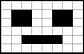## 2016 +

There are two main types of graphics used in computer systems: raster (also known as bitmap) and vector graphics.

## Vector Graphics

Raster graphics are made up of a grid of pixels.Vector graphics use objects (lines and curves) to mathematically form shapes.

If scaled to a larger size, a vector graphic does not lose any image quality.

If scaled to a larger size, a raster graphic loses image quality.

Raster graphics are generally larger in file size because data is stored for each pixel.

Vector graphics are generally smaller in file size.

Examples of raster images include photographs and screenshots.Examples of vector graphics include logos and cartoons.

## File Size = Resolution x Colour Depth

The resolution of an image is the width in pixels multiplied by the height in pixels.

The colour depth (also known as bit depth) is the number of bits that are used to represent each pixel's colour.

1 bit represents 2 colours (0 or 1 / black or white). 2 bits will allow for 4 colours, 3 bits for 8 colours, 4 for 16 etc. A colour depth of 1 byte (8 bits) allows for 256 different colours

Remember you must multiply the colour depth, not the number of available colours (e.g. 8 not 256).

The RGB (Red, Green, Blue) colour model uses 3 bytes (a byte of 256 red shades, a byte of 256 green shades and a byte of 256 blue shades) that together can represent 16.7 million different colours.

## 6 bits## Width = 8 bits

Look carefully at the exam question to see if the examiner is expecting the answer in bits, bytes or kilobytes.

Always calculate the file size in bits first then:

• Divide the file size in bits by 8 to convert to bytes.

• Divide the file size in bytes by 1000 to convert to kilobytes.

• Dimensions

• Colour depth

• Make

• Model

• Orientation

• Exposure time

Metadata is important, For example, the dimensions must be known so the image can be displayed correctly.## Questo's Questions

4.4 - Graphical Representation:

1. Describe three differences between raster (bitmap) and vector images. 

2. How many colours can be represented with a colour depth of...

• a. 1 bit 

• b. 5 bits 

• c. 1 byte 

3. How is the file size of an image calculated? 

4a. An image file has a width of 10 pixels, a height of 8 pixels and a colour depth of 2. What is the file size in bytes? 

4b. An image file has a width of 120 pixels, a height of 120 pixels and a colour depth of 1. What is the file size in kilobytes? 

4c. An image file has a width of 32 pixels, a height of 21 pixels and a colour depth of 1. What is the file size in bytes? 

5. State what is meant by metadata and give three examples of metadata for a graphics file.width in pixels, e.g. 720

height in pixels,
e.g. 480